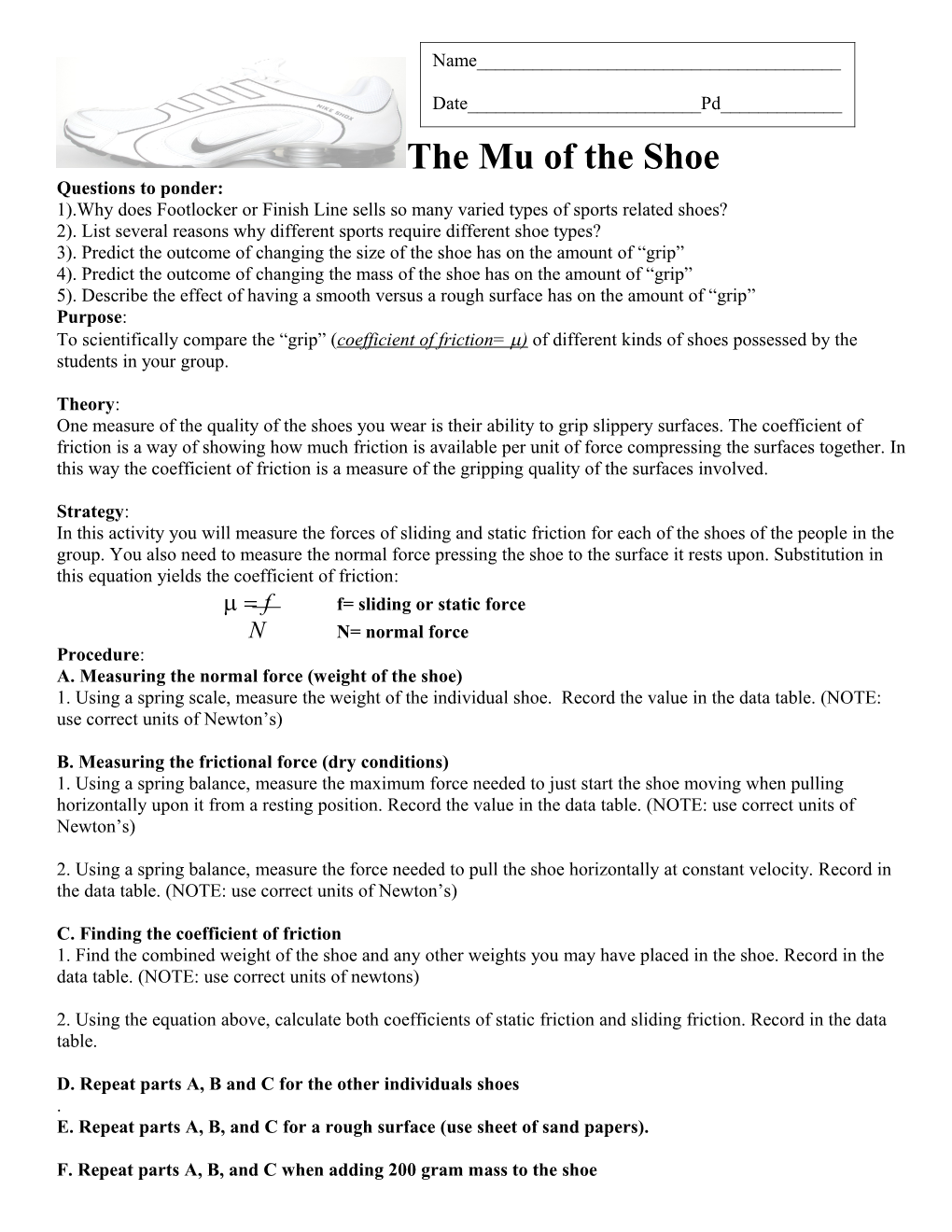# The Mu of the ShoeThe Mu of the Shoe

Questions to ponder:

1).Why does Footlocker or Finish Line sells so many varied types of sports related shoes?

2). List several reasons why different sports require different shoe types?

3). Predict the outcome of changing the size of the shoe has on the amount of “grip”

4). Predict the outcome of changing the mass of the shoe has on the amount of “grip”

5). Describe the effect of having a smooth versus a rough surface has on the amount of “grip”

Purpose:

To scientifically compare the “grip” (coefficient of friction= ) of different kinds of shoes possessed by the students in your group.

Theory:

One measure of the quality of the shoes you wear is their ability to grip slippery surfaces.The coefficient of friction is a way of showing how much friction is available per unit of force compressing the surfaces together. In this way the coefficient of friction is a measureof the gripping quality of the surfaces involved.

Strategy:

In this activity you will measure the forces of sliding and static friction for each of theshoes of the people in the group. You also need to measure the normal force pressing theshoe to the surface it rests upon. Substitution in this equation yields the coefficient offriction:

ff= sliding or static force

N N= normal force

Procedure:

A. Measuring the normal force (weight of the shoe)

1. Using a spring scale, measure the weight of the individual shoe. Record the value in the data table. (NOTE: use correct units of Newton’s)

B. Measuring the frictional force (dry conditions)

1. Using a spring balance, measure the maximum force needed to just start theshoe moving when pulling horizontally upon it from a resting position.Record the value in the data table. (NOTE: use correct units of Newton’s)

2. Using a spring balance, measure the force needed to pull the shoehorizontally at constant velocity. Record in the data table. (NOTE: use correct units of Newton’s)

C. Finding the coefficient of friction

1. Find the combined weight of the shoe and any other weights you may haveplaced in the shoe. Record in the data table. (NOTE: use correct units of newtons)

2. Using the equation above, calculate both coefficients of static friction andsliding friction. Record in the data table.

D. Repeat parts A, B and C for the other individuals shoes

.

E. Repeat parts A, B, and C for a rough surface (use sheet of sand papers).

F. Repeat parts A, B, and C when adding 200 gram mass to the shoe

Data Table A Smooth Surface

Brand/ Types of shoe / Static Friction Force (N) / Sliding Friction Force (N) / Normal Force (N) / Coefficient of Static Friction / Coefficient of Sliding Friction

Data Table B Rough Surface

Brand/ Types of shoe / Static Friction Force (N) / Sliding Friction Force (N) / Normal Force (N) / Coefficient of Static Friction / Coefficient of Sliding Friction

Data Table C 200 gram Mass

Brand/ Types of shoe / Static Friction Force (N) / Sliding Friction Force (N) / Normal Force (N) / Coefficient of Static Friction / Coefficient of Sliding Friction

Analysis Questions:Answer on separate sheet of paper

1. Which shoe had the strongest grip under smooth conditions?

2. Which shoe had the strongest grip under rough conditions?

3. Make a bar graph that compares the coefficients of static and sliding friction forthe brands of shoes that you tested. (NOTE: be sure to correctly label all parts of your graph and include title and key)

4. Explain whether the coefficient of static friction or the coefficient of sliding frictionbest applies to the situation of walking across the floor.

5. What other qualities besides the grip would a consumer want to have in the shoe theywant to buy?

6. Pick out one other quality (from question 5) and describe how you couldscientifically test for it in a side by side comparison of different brands.

7. Identify a sport and changing weather situation that would cause the athlete to want to increase friction to have better footing. Name the sport describe the change in weather conditions and finally what the athlete would have to do to the shoe to increase friction?

8. Identify a sport where an athlete wants to have frictional forces at a minimum.